# PSK and QAM Modulation Schemes

Daniel Weibel
Created 5 Feb 2016

Illustration of the different types of Phase-Shift Keying Modulation (PSK) and Quadrature Amplitude Modulation (QAM) schemes.

# PSK Modulation Schemes

The distinct states of the output signal are achieved by varying the phase of the carrier signal.

## Binary PSK (BPSK)

• Other names:
• 2-PSK
• 2 distinct phases $\rightarrow$ 2 symbols $\rightarrow$ 1 bit/symbol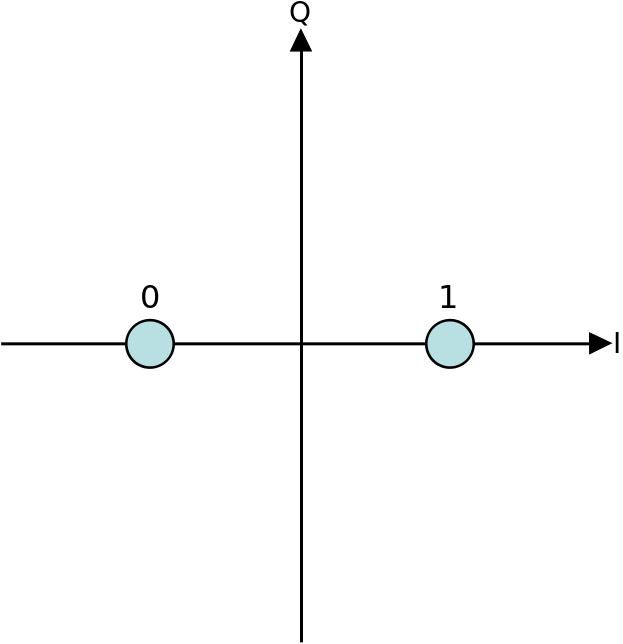• Other names:
• 4-PSK
• 4-QAM
• 4 distinct phases $\rightarrow$ 4 symbols $\rightarrow$ 2 bits/symbol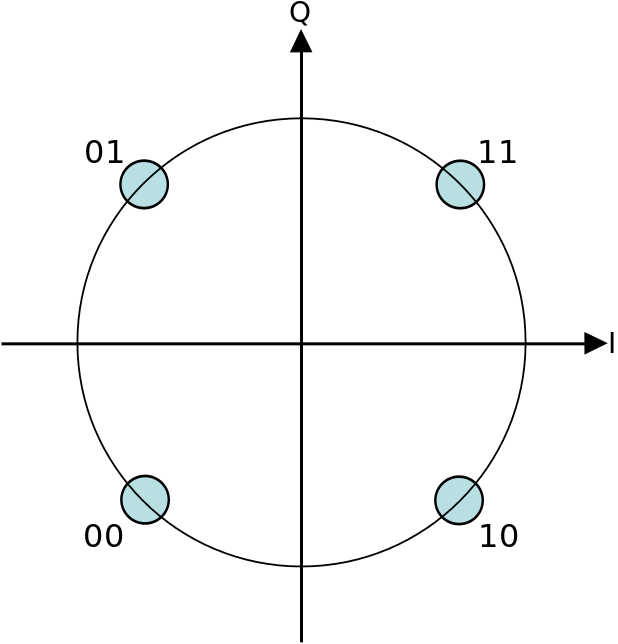• 4-PSK and 4-QAM are based on different concepts, however, they result in the same modulated signal.
• Used in LTE

## 8-PSK

• 8 distinct phases $\rightarrow$ 8 symbols $\rightarrow$ 3 bits/symbol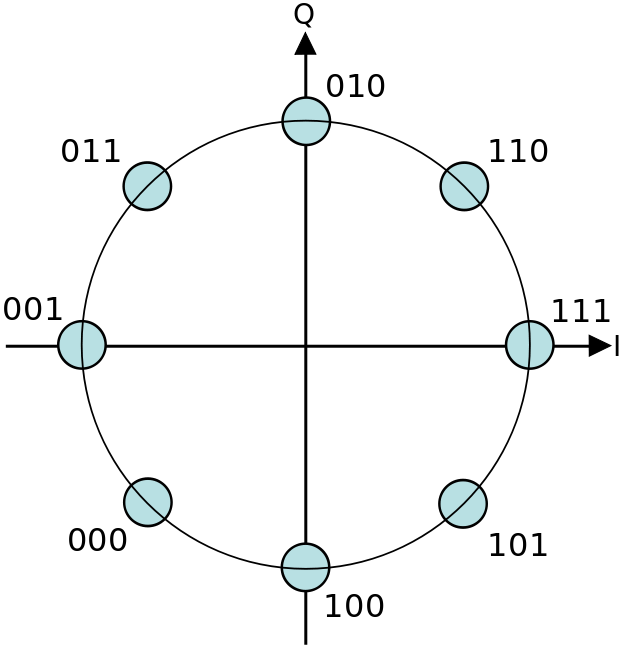• Highest-order PSK modulation scheme that is used in practice. In e.g. 16-PSK, with 16 distinct phases, the error rate would be too high. If a higher bits/symbol rate is needed, then another modulation scheme (such as QAM) is used.

# QAM Modulation Schemes

Combination of phase-shift keying (PSK) and amplitude-shift keying (ASK). The distinct states of the ouptut signal are achieved by the combinations of a number of distinct phases and a number of distinct amplitudes.

## 4-QAM

• 4 phases $\times$ 1 amplitude $\rightarrow$ 4 symbols $\rightarrow$ 2 bits/symbol• In effect, identical to QPSK

## 16-QAM

• E.g.: 8 phases $\times$ 2 amplitudes $\rightarrow$ 16 symbols $\rightarrow$ 4 bits/symbol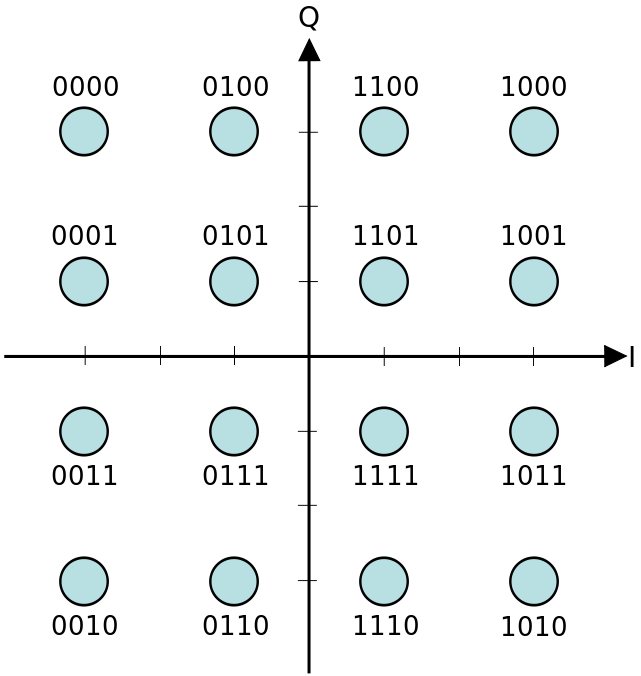• Other combinations of phases and amplitudes are possible. There must be at least 16 combinations to accommodate the 16 symbols.
• Used in LTE

## 64-QAM

• E.g.: 16 phases $\times$ 4 amplitudes $\rightarrow$ 64 symbols $\rightarrow$ 6 bits/symbol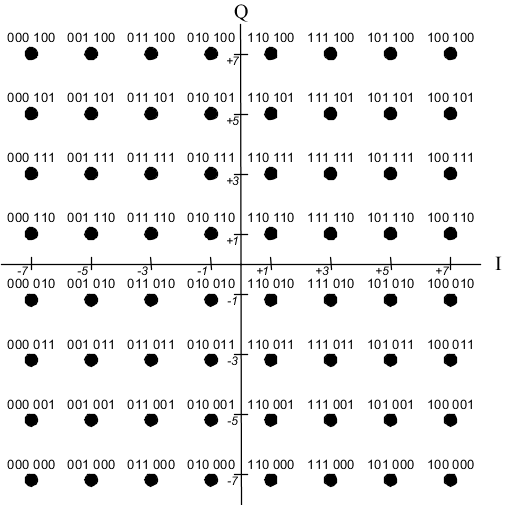• Other combinations of phases and amplitudes are possible. There must be at least 64 combinations to accommodate the 64 symbols.
• Used in LTE

## 256-QAM

• E.g.: 32 phases $\times$ 8 amplitudes $\rightarrow$ 256 symbols $\rightarrow$ 8 bits/symbol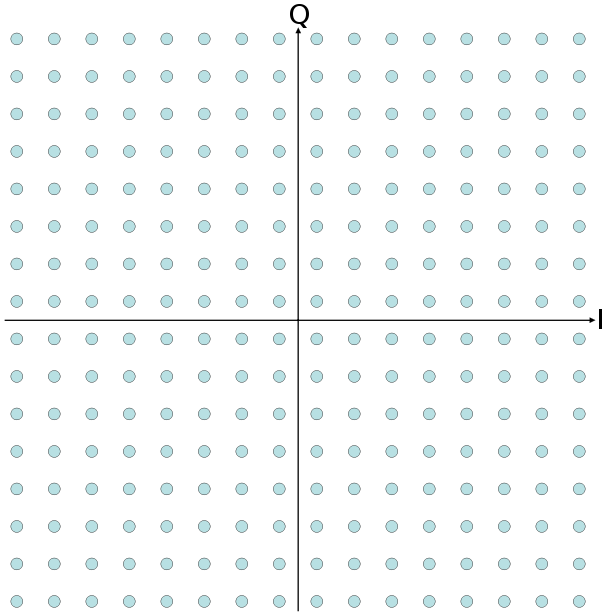• Other combinations of phases and amplitudes are possible. There must be at least 256 combinations to accommodate the 256 symbols.